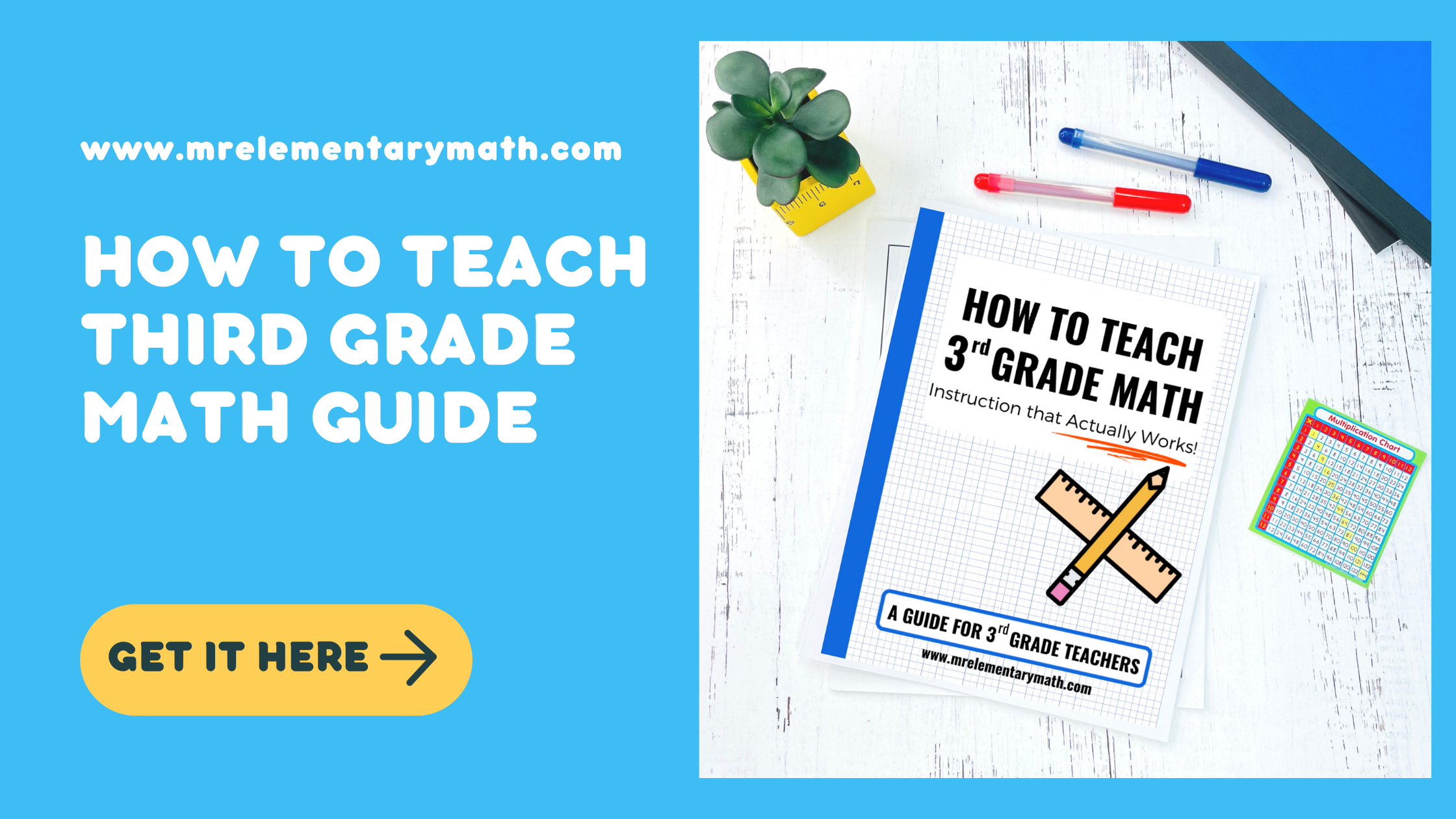Take the stress out of implementing math centers in your classroom...

## Make Teaching 3rd Grade Math Less Intimidating

This FREE guide is filled with helpful information, engaging ideas and relevant resources for teaching 3rd grade math.

# The Essentials: How to Teach 3rd Grade Math

Are you a new third grade teacher and want to know how to teach 3rd grade math? Or are you a vet that just needs a refresher on the important skills for the grade level? If this is you, save this post so that you can refer back to it throughout the school year.

It’s helpful to have an overview of what your students will be working towards during the school year all in one place.

##### So,let’s get to it! The 5 main domains taught in 3rd grade are:
• Operations and Algebraic Thinking
• Base Ten Numbers
• Fractions
• Measurement and Data
• Geometry

To help you out, I’ll give a brief overview of each one.

## Teaching Operations and Algebraic Thinking

The first domain is rooted in the 4 basic operations: addition, subtraction, multiplication, and division. Over time, students build their understanding of the properties of arithmetic and use this knowledge to solve real-world and mathematical problems.

### Multiplication and Division

In third grade, students shift from using repeated addition to find the total number of objects to using multiplication to find the products of whole numbers.

They are also introduced to the concept of division using two different models (partition model and measurement model). Third graders are also asked to describe the relationship between multiplication and division.

Throughout the school year, students use manipulatives, draw math models, and write equations to solve problem situations requiring multiplication and division.

##### Here are some of the sub-skills students practice and develop in 3rd grade:
• Multiply and divide within 100
• Understand properties of multiplication (i.e. distributive, commutative, etc.)
• Represent and solve problems involving multiplication and division
• Understand the relationship between multiplication and division

### Arithmetic Patterns

Students are expected to be able to identify and explain patterns involving arithmetic with whole numbers.

##### Examples include finding patterns using math tools like:
• multiplication tables
• hundreds charts

### Multi-Step Word Problems

In 3rd grade, word problem solving expands from one and two-step word problems that involve addition and subtraction to two-step problems using all four operations.

## TeachingBase Ten Numbers

The second domain is Base Ten Numbers. In third grade, students use their understanding of place value to extend their knowledge of larger numbers and are expected to fluently solve addition and subtraction problems within 1,000. In some states, this number extends to 10,000.

##### Strategies for solving these problems include:
• place value
• properties of operations
• the relationship between addition and subtraction

In addition to adding and subtracting numbers, students also begin to use math models like number lines to develop an understanding of rounding numbers to the nearest 10 and 100.

## Teaching Fractions

The next domain is fractions. As 3rd graders continue to investigate equal parts of a whole, they begin to discover the size of a fractional part is dependent on the size of the whole. As they partition segments into equal parts, they learn about denominators and numerators.

In addition, students use math models like number lines and fraction strips to develop a conceptual understanding of a fraction as a number in relation to a defined whole. Third graders typically explore a limited number of fractions. They work with fractions that have the following denominators: 2, 3, 4, 6, and 8.

##### Here are some of the sub-skills students practice and develop in 3rd grade:
• develop understanding of fractions as numbers
• compare fractions with like denominators
• understand equivalent fractions
• partition visual models

## Teaching Measurement and Data

The fourth domain is Measurement and Data. In 3rd grade, the study of measurement ties directly to students’ daily lives.

### Time

Students learn how to tell and write time to the nearest minute. They also begin to solve word problems with elapsed time using models and tools like clock models and number lines.

### Measurement

Third-grade students learn to become proficient in using tools to measure liquid volume and mass. In addition, they begin solving word problems that involve mass and liquid volume.

### Area and Perimeter

Third graders explore area measurement. They begin by investigating the concept of measuring area by covering a figure with unit squares and then counting to determine the area of the surface. The learning later extends to relating the area of a rectangle to multiplication and finding the area of composite and rectilinear shapes.

In addition, students find the perimeter of unknown side lengths of polygons. They also compare the area and perimeter of different shapes.

##### Here are some of the sub-skills students practice and develop in 3rd grade:
• Understand the concept of area
• Relate area to multiplication and addition
• Find the area of composite and rectilinear shapes
• Understand the concept of perimeter
• Distinguish between area and perimeter

### Data

Students create, read and analyze picture graphs, bar graphs, and line plots. In addition, they’re expected to use the information in the graphs to solve problems.

## Teaching Geometry

The last domain is Geometry. Third graders continue to identify, draw and classify different polygons like triangles, pentagons, and hexagons. In addition, students take a deeper dive to investigate quadrilaterals and their attributes.

And there you have it! A complete overview of the essential standards for third-grade math.

## Grab my FREE Guide, How to Teach 3rd Grade Math: Instruction that Actually Works3